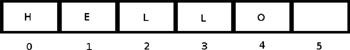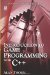# 6.11 Data and Arrays

## 6.11 Data and Arrays

A former teacher of mine always used to repeat the same old joke to his students each term. "There are three types of arrays," he said. "A one-dimensional array, a two-dimensional array, and a hip-hip-array." One thing is for certain: He was correct about the first two kinds of arrays. In C++, an array is a sequential collection of data in memory. This section examines them more closely. Consider Figure 6.2, which illustrates an array in memory.Figure 6.2

### 6.11.1 Array Definition

An array is a sequential collection of variables or constants. For example, to store the age of a person I can declare an integer variable. If I then asked 100 people their age and used them in a program, I would need 100 separate variables-one to hold each age. Declaring 100 single variables like this, each with unique names, would indeed be quite a tiresome task. This problem can be solved using arrays because an array is a list, and so is considered to be a list of variables. Consider the following code:

`      #include <iostream>      int main()      {         int Num_Array;         Num_Array = 10;         return 0;      } `

### 6.11.2 Array Declaration

Arrays can generally be declared in one of two forms:

• DataType ArrayName [Size of Array];`      int Numbers; `Here, the array is declared and an appropriate amount of memory is reserved for 10 numbers. Each of these 10 numbers is initially set to the value 0.

• DataType ArrayName [] = {Value1, Value2, Value3, }`      int Numbers[] = {1, 2, 3, 4, 5}; `Here, the array is declared and sized to hold five numbers, which are initially set to the values of 1, 2, 3, 4, and 5.

 Note When a variable, constant, array, or any data storage is assigned values for the first time, it is said to be initialized.

### 6.11.3 Array Usage

Each item in an array is called an element, and every element has a position in the array, called its index. So, the first element in an array has an index of 0, the next an index of 1, the next 2, and so on. In order to access an element, the subscript operator ([]) is used. It takes the following form:

• ArrayName [Index];

The following sample code declares an array and demonstrates how sample values can be assigned to array elements in the same way normal variables can be given assignments.

 Warning The compiler performs no error checking with regard to array indexes. If a programmer attempts to access an array element that does not exist, the program will compile and when this code is encountered during execution, there will be a run-time error.

For example:

`      int Ages;      Ages = 10; //Is not valid because there is no element 33 `

### 6.11.4 Two-dimensional Arrays

The arrays considered thus far have been one dimensional; in other words, they are a linear list of items stored sequentially in memory. However, arrays need not be restricted to one dimension. They can also be two dimensional. Data organized in a 2D array can be thought of as being arranged in a grid with columns and rows. Two-dimensional arrays take the following declaration form:

• DataType ArrayName [rows] [columns];`      int Numbers  ; `2D arrays can be accessed in the same ways as 1D arrays, but programmers should be sure to acknowledge the extra dimension. Consider the following sample:

`      Numbers = 257; `
 Note Arrays can extend to as many dimensions as a programmer needs.Introduction to Game Programming with C++ (Wordware Game Developers Library)
ISBN: 1598220322
EAN: 2147483647
Year: 2007
Pages: 225
Authors: Alan Thorn

Similar book on Amazon

flylib.com © 2008-2017.
If you may any questions please contact us: flylib@qtcs.net